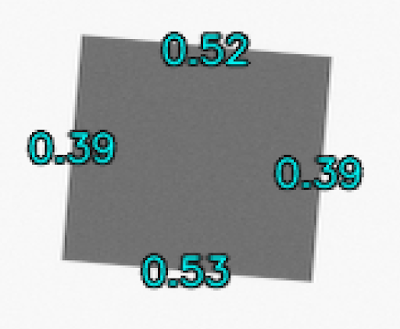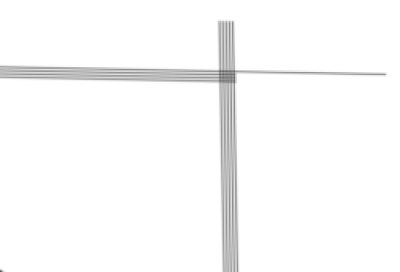## Tuesday, 1 August 2017

### Image interpolation: Fighting the fade, part 1

Over the last six months or so I kept on bumping into a particularly vexing problem related to image interpolation: the contrast in an interpolated image drops to zero in the worst case of interpolating at an exact half-pixel shift relative to the original image.

Consider the case where you are trying to co-register (align) two images, such as two images captured by the same camera, but with a small translation of the camera between the two shots. If we translate the camera purely in the horizontal direction, then the shift between the two images will be h pixels, where h can be any real number. The integer part of h will not cause us any trouble for reasonable values of h, such that the two images still overlap, of course. The trouble really lies in the fractional part of h, since this forces us to interpolate pixel values from the moving image if we want it to line up correctly with the fixed image.

The worst case scenario, as mentioned above, is if the fractional part of h is exactly 0.5 pixels, since this implies that the value of a pixel in the interpolated moving image will be the mean of the two closest pixels from the original moving image. Figure 1 illustrates what such an interpolated moving image will look like for a half-pixel shift; the edges are annotated with their MTF50 values.Figure 1: Scaled (Nearest Neighbour interpolation) view of an interpolated moving image that experienced a half-pixel shift. The numbers are the measured MTF50 values, in cycles/pixel.
Looking closely at the vertical edges of the gray square, we can see that there are some visible interpolation artifacts manifesting as overshoot and undershoot. This image was interpolated using OMOMS cubic spline interpolation , which is best method that I am aware of. Linear interpolation would produce much more blurring (but no overshoot/undershoot). And of course we see a marked drop in MTF50 on the vertical edges!

At any rate, the MTF curves for the edges are illustrated in Figure 2. The blue curve corresponds to the horizontal edge (i.e., the direction that experienced no interpolation), and the orange curve corresponds to the vertical edge (an 0.5-pixel horizontal shift interpolation). The green curve was obtained from another simulation where the moving image was shifted by 0.25 pixels.Figure 2: MTF curves of interpolated moving images corresponding to fractional horizontal shifts of zero (blue), 0.25 pixels (green), and 0.5 pixels (orange).

Certainly the most striking feature of the orange curve is how the contrast drops to exactly zero at the Nyquist frequency (0.5 cycles/pixel). The smaller 0.25-pixel shift (green curve) shows a dip in contrast around Nyquist, but this would probably not be noticeable in most images.

In Figure 3 we can see that this loss of contrast around Nyquist follows a smooth progression as we approach a fractional shift of 0.5 pixels.Figure 3: MTF curves of interpolated moving images corresponding to fractional horizontal shifts of 0.25 pixels (blue), 0.333 pixels (green), and 0.425 pixels (orange).
The conclusion from this experiment is that we really want to avoid interpolating an image with a fractional shift of 0.5 pixels (in either/both horizontal and vertical directions), since this will produce a very noticeable loss of contrast at higher frequencies, i.e., we will lose all the fine details in the interpolated image.

An applied example of where this interpolation problem crops up is when we apply a radial distortion correction model to improve the geometry of images captured by a lens exhibiting some distortion (think barrel or pincushion distortion). I aim to write a more thorough article on this topic soon, but for now it suffices to say that our radial distortion correction model specifies for each pixel (x, y) in our corrected image where we have to go and sample the distorted image.

I prefer to use the division model , which implies that for a pixel (x, y) in the corrected image, we go and sample the pixel at
x' = (x - xc) / (1 + k1r2 + k2r4) + xc
where
r = sqrt((x - xc)2 + (y - yc)2)
and (xc, yc) denotes the centre of distortion (which could be the centre of the image, for example).
The value of y' is calculated the same way. The actual distortion correction is then simply a matter of visiting each pixel (x, y) in our undistorted image, and setting its value to the interpolated value extracted from (x', y') in the distorted image.

The important part to remember here is that the value (x', y') can assume any fractional pixel value, including the dreaded half-pixel shift.

### An example of mild pincushion distortion

In order to illustrate the effects of radial distortion correction, I thought it best to start with synthetic images with known properties. Figure 4 illustrates a 100% crop near the top-left corner of the reference image, i.e., what we would have obtained if the lens did not have any distortion.Figure 4: The pure, undistorted reference image. Note that the closely-spaced black lines blur into gray bars because of the simulated Gaussian Point Spread Function (PSF) with an MTF50 of 0.35 c/p. If you squint hard enough, you can see some traces of the original black bars. Rendered at 400% size with nearest-neighbour upscaling. (click for 100% view)

I simulated a very mild pincushion distortion with k1 = 0.025 and k2 = 0, which produces an SMIA lens distortion figure of about -1.62%. This distortion was applied to the polygon geometry, which was again rendered with a Gaussian PSF with an MTF50 of 0.35 c/p. The result is shown in Figure 5. Keep in mind that you cannot really see the pincusion distortion at this scale, since we are only looking at the top-left corner of a much larger image.Figure 5: Similar to Figure 4, but with about 1.62% pincushion distortion applied to the polygon geometry. Rendered at 400% size with nearest-neighbour upscaling. (click for 100% view)

We can see the first signs of trouble in Figure 5: Notice how the black/white bars appear to "fade out" at regular intervals. The straight lines of Figure 4 are no longer perfectly straight, nor are they aligned with the image rows and columns. The lines thus cross from one row (or column) to the next, and the gray patches correspond to the regions where the lines fell halfway between two rows (or columns), leading to the apparent loss of contrast.

It is important to understand at this point that the fading in Figure 5 is not a processing artifact; this is exactly what would happen if you were to photograph similar thin bars that are not aligned with the image rows/columns.

Finally, we arrive at the radial distortion correction phase. Figure 6 illustrates what the corrected image would look like if we used standard cubic interpolation to resample the image.Figure 6: The undistorted version of Figure 5. Resampling was performed using standard cubic interpolation. Rendered at 400% size with nearest-neighbour upscaling. (click for 100% view).
We see some additional fading that appears in Figure 6. If you flick between Figures 5 and 6 (after clicking for 100% view) you will notice that an extra set of fading patches appear in between the original fading patches. These extra fades are the manifestation of the phenomenon illustrated in Figure 2: the contrast drops to zero as the interpolation sample position approaches a fractional pixel offset of 0.5. The interesting thing about these additional fades is that they are not recoverable using sharpening --- once the contrast reaches zero, no amount of sharpening will be able to recover it.

### A potential workaround

The aim of radial distortion correction is to remove the long range (or large scale) distortion, since the curving of supposedly straight lines (e.g., building walls) is only really visible once the distortion produces a shift of more than one pixel. Unfortunately we cannot simply ignore the fractional pixel shifts --- this would be equivalent to using nearest-neighbour interpolation, with its associated artifacts.

Perhaps we can cheat a little: what if we pushed out interpolation coordinates away from a fractional pixel shift of 0.5? Let x' be the real-valued x component of our interpolation coordinate obtained from the radial distortion correction model above. Further, let xf be the largest integer less than x' (the floor of x'). If x' - xf < 0.5, then let d = x' - xf. (We can deal with the d > 0.5 case by symmetry).

Now, if d > 0.375, we compress the value of d linearly such that 0.375 <= d' <= 0.425. We can obtain the new value of x', which we can call x", such that x" = xf + (x' - xf ) * 0.4 + 0.225. Looking back at Figure 3, we see that a fractional pixel shift of 0.425 seems to leave us with at least a little bit of contrast; this is where the magic numbers and thresholds were divined from.

Does this work? Well, Figure 7 shows the result of the above manipulation of the interpolation coordinates, followed by the same cubic interpolation method used in Figure 6.Figure 7: The undistorted version of Figure 5. Resampling was performed using the modified interpolation coordinates followed by cubic interpolation. Rendered at 400% size with nearest-neighbour upscaling. (click for 100% view).
Careful squinting reveals that the additional fading patches observed in Figure 6 have been reduced noticeably. This looks promising. Of course, one might argue that I have just added some more aliasing to the image. Which might be the case.

Further testing will be necessary, especially on more natural looking scenes. I might be able to coax sufficient distortion from one of my lenses to perform some real-world experiments.

### Further possibilities

Using the forced geometric error method proposed above, we can now extract at least some contrast at the frequencies near Nyquist. We also know what the fractional pixel shift was in both x and y, so we know what the worst-case loss-of-contrast would be. By combining these two bits of information we can sharpen the image adaptively, where the sharpening strength is adjusted according to the expected loss of contrast.

Stay tuned for part two, where I plan to investigate this further.

### References

1. Fitzgibbon, A.W.: Simultaneous linear estimation of multiple view geometry and lens distortion. In: Proc. IEEE International Conference on Computer Vision and Pattern Recognition, pp. 125–132 (2001).
2. Thevenaz, P., Blu T. and Unser, M.: Interpolation revisited, IEEE Transactions on medical imaging, 19(7), pp. 39–758, 2000.